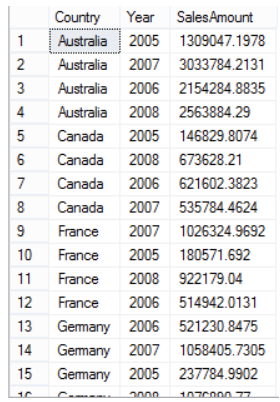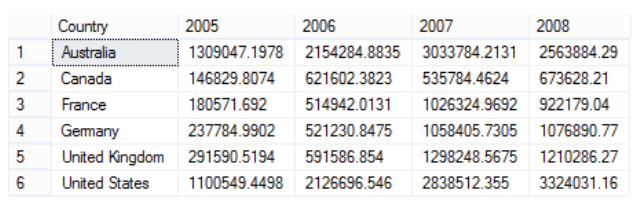# Pivot Queries

Contents

Overview

## Overview

In data processing, a pivot table can automatically sort, count, total or give the average of the data from a table, displaying the results in a second table showing the summarized data. Pivot tables are also useful for quickly creating unweighted cross tabulations. LiveView supports pivot queries to create pivot tables.

In LiveView, you can select a column in the source table as a pivot column and create columns in a destination table for each value in the source pivot column.

The following LiveView clients support pivot queries:

 LiveView Web 1.2 and higher JavaScript Client API Java Client API lv-client

In the following example, the pivot operation converts different values in the column `year` to columns in the destination table. An aggregate expression then evaluates the data of every pivot column data point for each group. Next, a summation on the `SalesAmount` is performed in the following `CountrySales` table in LiveView for the years 2005, 2006, 2007, and 2008.The GROUP BY operation is performed on some columns. In this example, GROUP BY is performed on the column, `Country`.

Consider the following LiveView query:

```Select * from CountrySales
PIVOT sum(SalesAmount)
FOR Year VALUES [2005, 2006, 2007, 2008]
GROUP BY Country```

Once pivoted, the destination table looks like this:The above example shows a basic pivot operation. LiveView also supports complex pivot table functionality, including:

Multiple aggregate expressions for each pivot column value

Instead of using only a sum(salesamount), you can configure `m` expressions such as sum(salesamount), max(salesamount), and avg(salesamount). Each expression is evaluated for each year value in the VALUES clause (2005, 2006, 2007, and 2008). The total number of columns is derived from the number of aggregate expressions, multiplied by the total number of values in the VALUES clause. For example:

```Select * from CountrySales PIVOT sum(SalesAmount), max(SalesAmount), avg(SalesAmount)
FOR Year VALUES [2005, 2006, 2007, 2008] GROUP BY Country```
Multiple pivot operations

LiveView pivot tables support `n` value expressions that use multiple columns as pivot columns, unlike the static use of single pivot column values such as `Year` as shown in the example above.

```Select * from CountrySales PIVOT sum(SalesAmount), max(SalesAmount), avg(SalesAmount)
VALUES [salesAmount>200.0, Year=2005, Year=2006, Year=2007, Year=2008,
someOtherFieldInTable = 'someValue'] GROUP BY Country```

For these complex pivot options, the total number of columns is ( (m*n) + any GROUP BY columns ).

### Column Naming Rules

When there are `m` number of aggregate expressions in the PIVOT clause and `n` number of expressions in the VALUES clause from the pivot column, the total number of resultant columns is `m*n`. Each aggregate expression is computed for each pivot value from the VALUES clause. The column name is formed by concatenating the aggregate expression's alias (first part below) and values expression's alias (second part below) and generating the result. The symbol used for concatenation is '`_`'.

First part of column header (from the pivot aggregate expression)

If aggregate expressions are aliased, LiveView uses that alias to become the first part of column header in the result set.

If pivot aggregate expressions are not aliased, LiveView uses `expr_0, expr_1, expr_2...` in increments for subsequent aggregate expressions. For example:

```PIVOT sum(salesAmount) as total, max(salesAmount)
1) sum(salesAmount) as total -> total
2) max(salesAmount) -> expr_0```
Second part of column header (from the value expressions):

If the value expression is aliased, LiveView uses the alias name.

If the value expression is not aliased, LiveView uses the pattern `_incrementingCounter(_0, _1, _2)`. If the value is a literal, LiveView generates a valid column header by replacing all unacceptable characters to '`_`'.

If the value is a number, LiveView prepends an underscore '`_`'.

For example:

```FOR YEAR VALUES [2015, YEAR=2016, 2017 as seventeen, noyear]
1) 2015 -> _2015 (forms a valid alias part, after prep-ending a '_')
2) YEAR = 2016 -> _0 (_incrementingCounter pattern)
3) 2017 as seventeen -> seventeen (alias provided)
4) noyear -> noyear ('noyear' the pivot value is a valid column header)```

After LiveView generates the first part's alias of the column header from the aggregate expression and the second part's alias from the pivot value, LiveView concatenates the two parts: the first part + '`_`' + the second part.

For example:

```PIVOT sum(salesAmount) as total, max(salesAmount)
FOR YEAR VALUES [2015, YEAR=2016, 2017 as seventeen, noyear, YEAR=2018]```

The Result schema will have the following column headers:

```total_2015, total_0, total_seventeen, total_noyear, total_1, expr_0_2015,
expr_0_0, expr_0_seventeen, expr_0_noyear, expr_0_1

total_2015       ----> total + '_' + _2015(if there is more than one '_' symbol while joining valid
part names, they are considered as one)
total_0          ----> total + '_' + _0(first value expression without alias for the pivot expression)
total_seventeen  ----> total + '_' + seventeen
total_noyear     ----> total + '_' + noyear
total_1          ----> total + '_' + _1(second value expression without alias for the pivot expression)

expr_0_2015      ----> expr_0 + '_' + _2015
expr_0_0         ----> expr_0 + '_' + _0(first value expression without alias for the pivot expression)
expr_0_seventeen ----> expr_0 + '_' + seventeen
expr_0_noyear    ----> expr_0 + '_' + noyear
expr_0_1         ----> expr_0 + '_' + _1(second value expression without alias for the pivot expression)```

### Pivot Query Structure Details

Consider the following example to understand pivot queries.

```SELECT * from CountrySales
PIVOT sum(SalesAmount), max(SalesAmount), avg(SalesAmount)
FOR Year
VALUES [2005, 2006, Year=2007, 2008, someOtherFieldInTable = 'someValue']
GROUP BY Country```

Breaking down the above LiveQL pivot query syntax to understand its components:

`SELECT *`

Only the wildcard '`*`' is allowed in the projection, which implies a combination of GROUP BY fields and values from the pivot column that satisfy expressions in the VALUES clause.

`FROM [TableName]`

LiveView base table name. In the example above, TableName = `Country`.

```PIVOT sum(SalesAmount), max(SalesAmount), avg(SalesAmount)
FOR Year
VALUES [2005, 2006, Year=2007, 2008, someOtherFieldInTable = 'someValue']```

The pivot operation contains three parts:

1. PIVOT: List of valid StreamBase aggregate expressions. In this example, `sum`, `max`, and `avg`.

2. FOR: Column to be pivoted. This clause is optional if all the expressions inside `VALUES` clause are valid StreamBase test expressions and none of them are literals. In this example, `Year`.

3. VALUES: The values are valid StreamBase test expressions or literals. If the clause has literals, the `FOR` clause becomes mandatory. In the following example, the literal 2007 is interpreted as `YEAR=2007`. Thus all literals are converted to expressions as `pivotColumnMentionedInFOR=literalValue` and the `VALUES` clause is treated as a collection of Boolean expressions.

`GROUP BY [field].`

In this example, GROUP BY base table name, `Country`. See LiveQL Reference for more information regarding LiveQL syntax.

The following are additional examples to understand user input and the resulting schemas.

Example 1.

```    SELECT *
FROM Sales
PIVOT sum(SALES) AS TOTAL, max(SALES) AS HIGHEST
FOR YEAR
VALUES [ YEAR<=2014 AS OLD, 2015, 2016]
GROUP BY SALESPERSON;```

Result schema: SALESPERSON, TOTAL_OLD, TOTAL_2015, TOTAL_2016, HIGHEST_OLD, HIGHEST_2015, HIGHEST_2016

Example 2.

```SELECT *
FROM Sales
PIVOT sum(SALES) AS TOTAL, max(SALES) AS HIGHEST
VALUES [ YEAR=2014, YEAR=2015, YEAR=2016]
GROUP BY SALESPERSON;```

Result schema: SALESPERSON, TOTAL_0, TOTAL_1, TOTAL_2 HIGHEST_0, HIGHEST_1, HIGHEST_2

Example 3.

```SELECT *
FROM Sales
PIVOT sum(if(curr='EURO') then AMT*1.2 else AMT)
FOR YEAR
VALUES [ YEAR<=2014 AS OLD, 2015, 2016]
GROUP BY SALESPERSON;```

Result schema: SALESPERSON, expr_0_OLD, expr_0_2015, expr_0_2016